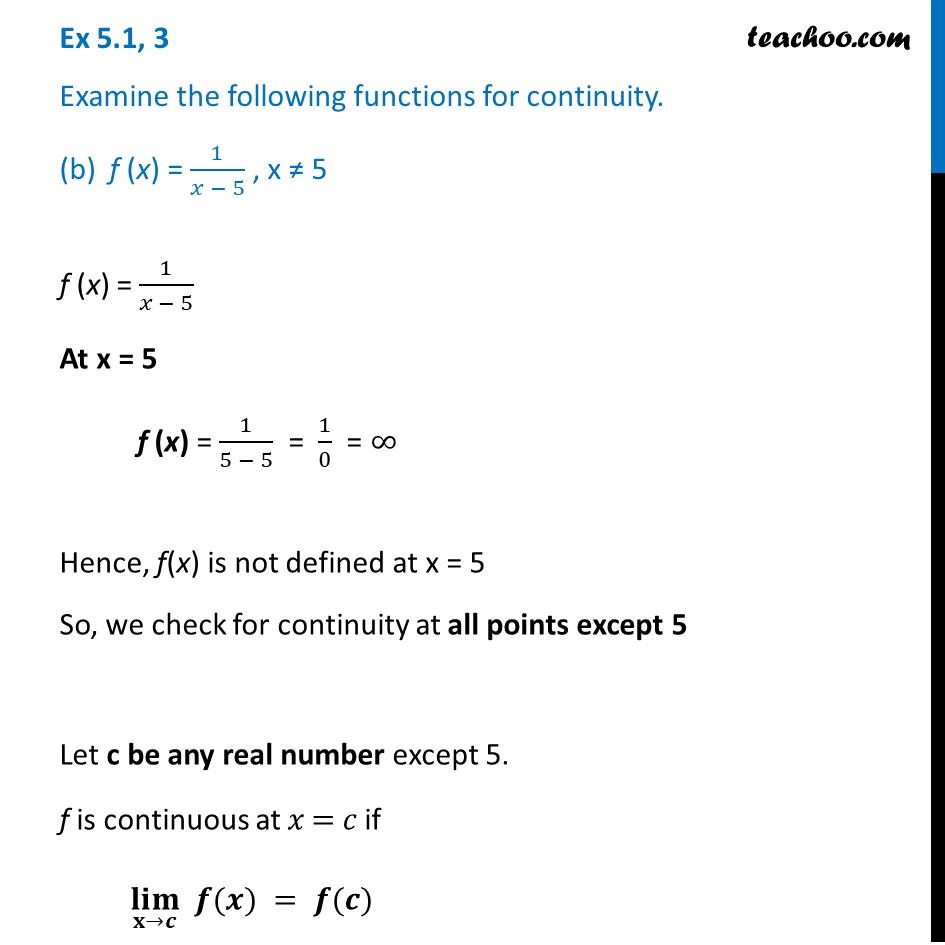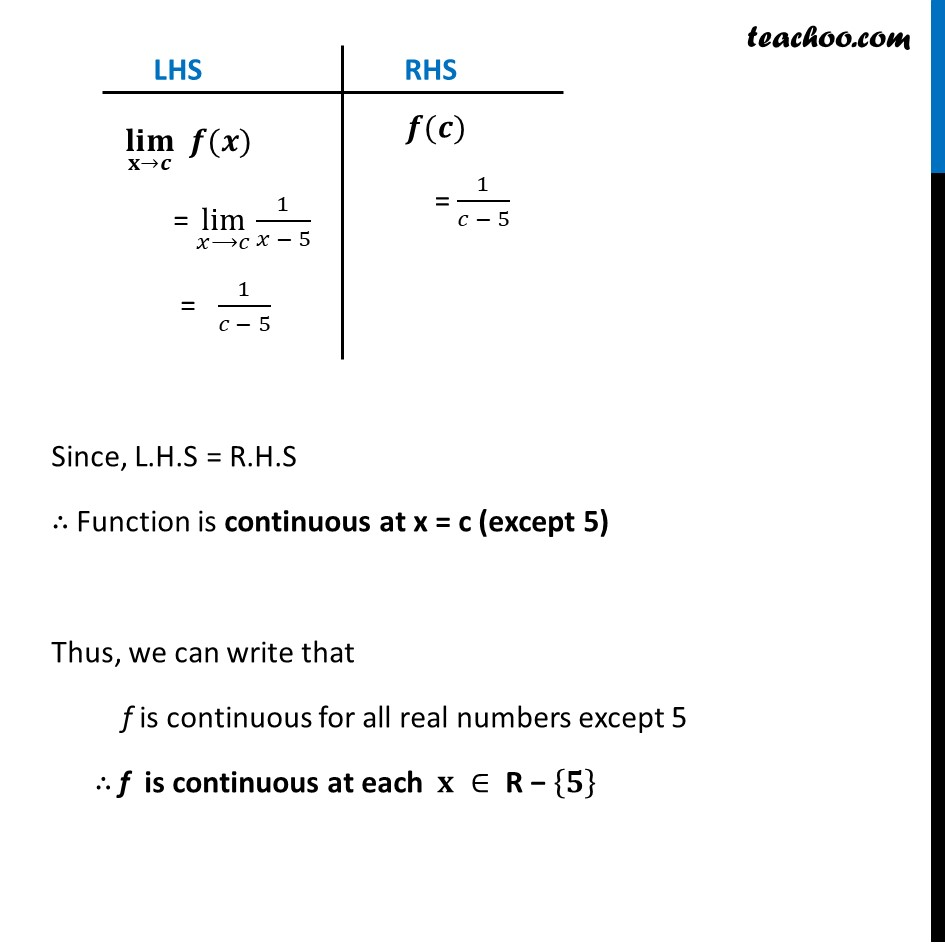Ex 5.1

Chapter 5 Class 12 Continuity and Differentiability
Serial order wiseLearn in your speed, with individual attention - Teachoo Maths 1-on-1 Class

### Transcript

Ex 5.1, 3 Examine the following functions for continuity. (b) f (x) = 1/(𝑥 − 5) , x ≠ 5 f (x) = 1/(𝑥 − 5) At x = 5 f (x) = 1/(5 − 5) = 1/0 = ∞ Hence, f(x) is not defined at x = 5 So, we check for continuity at all points except 5 Let c be any real number except 5. f is continuous at 𝑥 = 𝑐 if (𝐥𝐢𝐦)┬(𝐱→𝒄) 𝒇(𝒙) = 𝒇(𝒄) LHS (𝐥𝐢𝐦)┬(𝐱→𝒄) 𝒇(𝒙) = lim┬(𝑥⟶𝑐)⁡〖1/(𝑥 − 5)〗 = 1/(𝑐 − 5) RHS 𝒇(𝒄) = 1/(𝑐 − 5) Since, L.H.S = R.H.S ∴ Function is continuous at x = c (except 5) Thus, we can write that f is continuous for all real numbers except 5 ∴ f is continuous at each 𝐱 ∈ R − {𝟓}